## This calculator combines 10 key financial calculators into one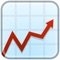# FinCal

by Karan Kumar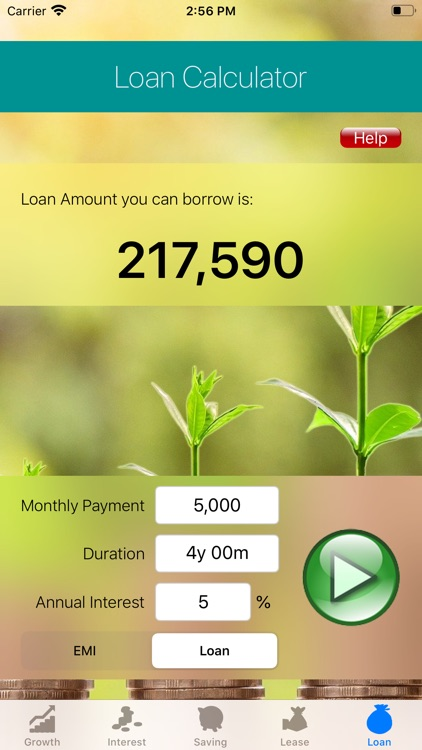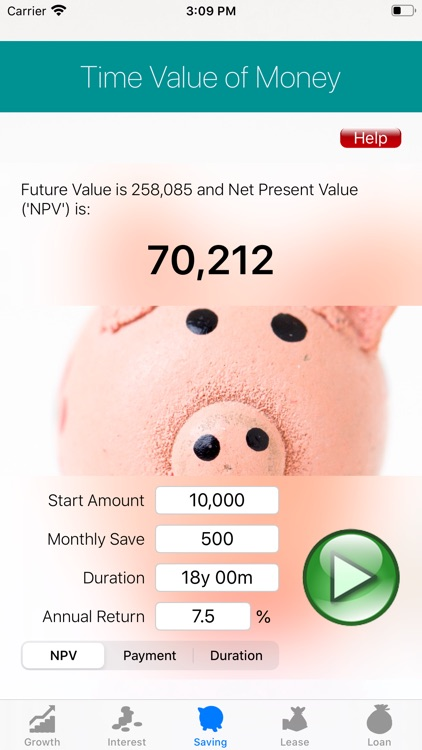This calculator combines 10 key financial calculators into one. These are:Version
1.1
Rating
(1)
Size
14Mb
Genre
Last updated
May 16, 2020
Release date
May 15, 2020

### App Screenshots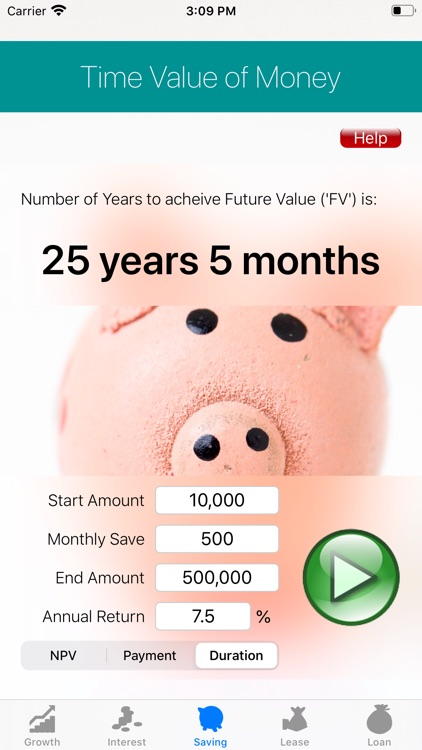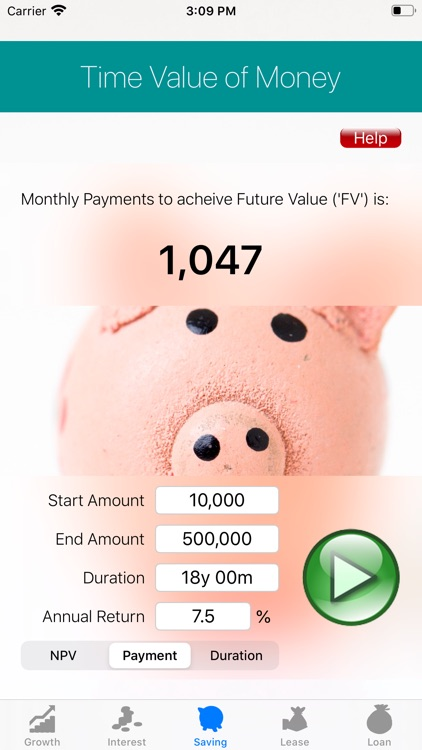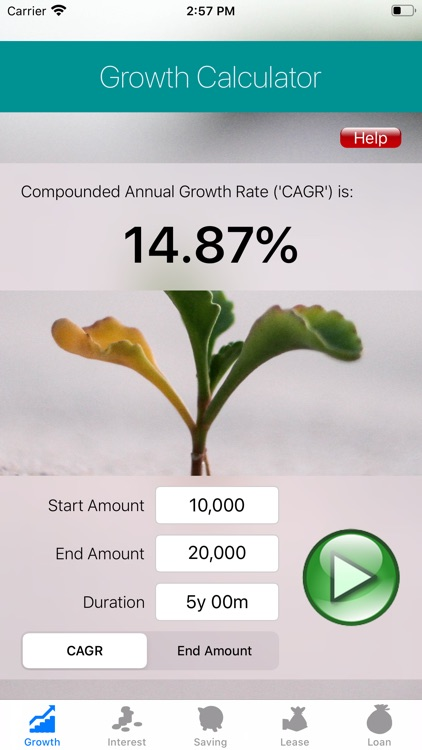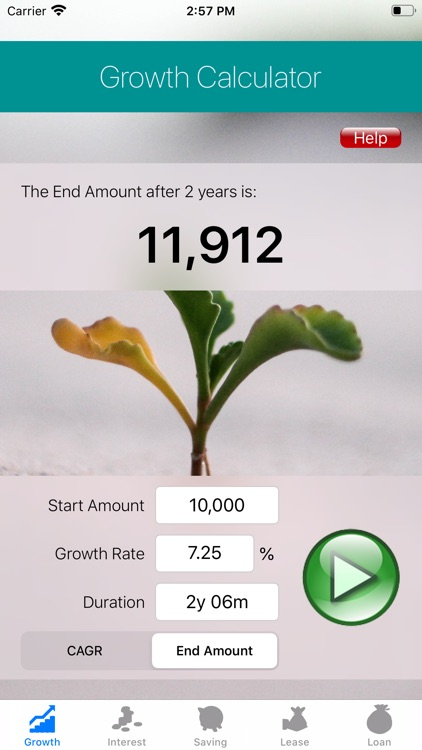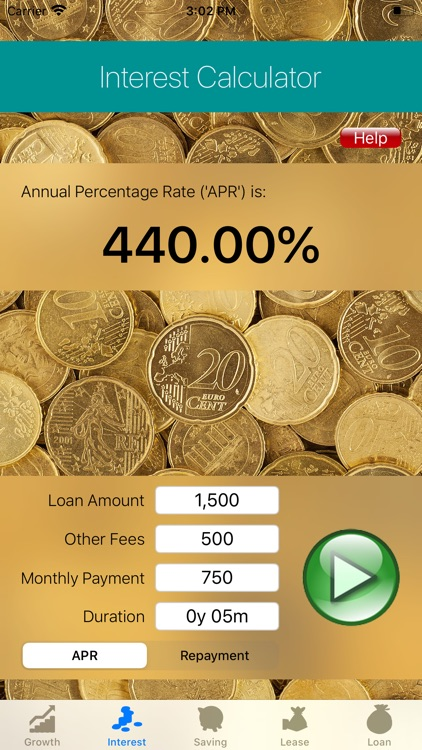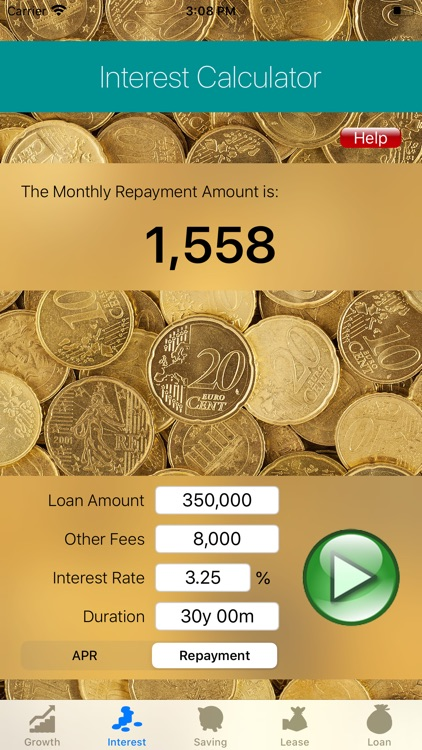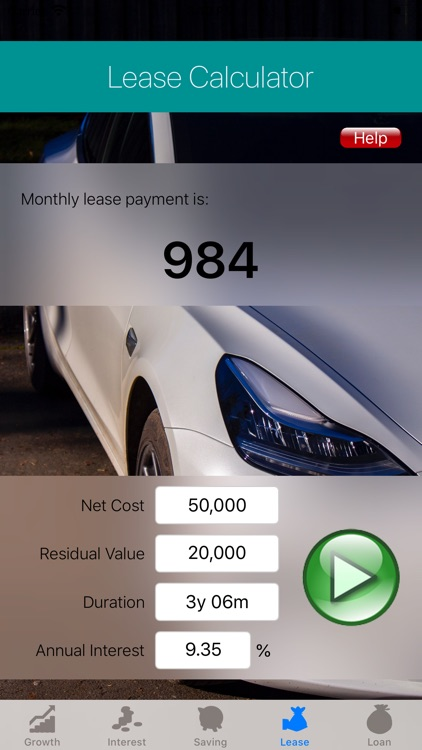### App Store Description

This calculator combines 10 key financial calculators into one. These are:

1. Compound Annual Growth Rate ('CAGR'): calculates the CAGR for given Start and End amounts.

2. Compounded End Amount: calculates the final (end) amount at the end of the period once a compounded growth rate has been applied.

3. Net Present Value ('NPV'): calculates the future value and NPV of the investment.

4. Future Value Payments: calculates the monthly payments required to achieve the defined end amount of investment.

5. Investment Duration: calculates the duration (in years and months) required for a Start Investment Amount to increase to a Target End Amount.

6. Lease Calculator: calculates the estimated monthly lease payments.

7. Loan Estimated Monthly Instalments ('EMI'): calculates the EMI payment for the loan.

8. Maximum Loan: calculates the maximum loan amount that can be borrowed for the defined monthly payment.

9. Annualised Percentage Rate ('APR'): calculates the APR applicable to the loan.

10. Loan Repayment: calculates the monthly repayment amount based on the defined APR.

Disclaimer:
AppAdvice does not own this application and only provides images and links contained in the iTunes Search API, to help our users find the best apps to download. If you are the developer of this app and would like your information removed, please send a request to takedown@appadvice.com and your information will be removed.